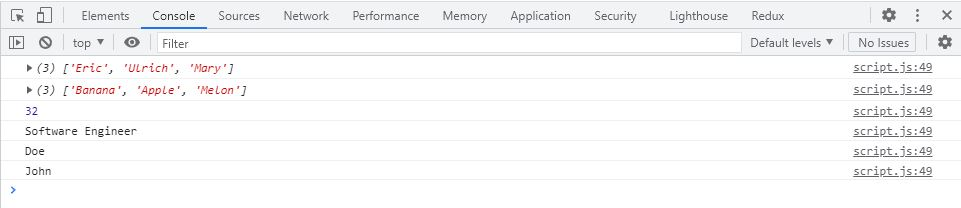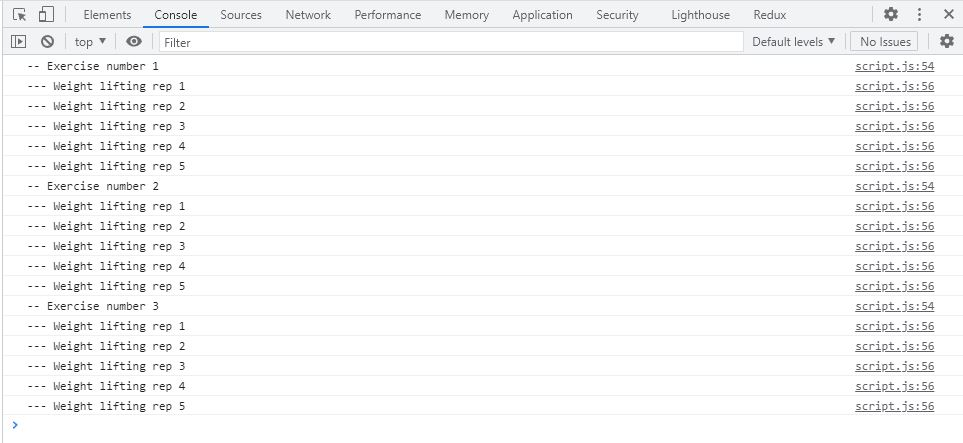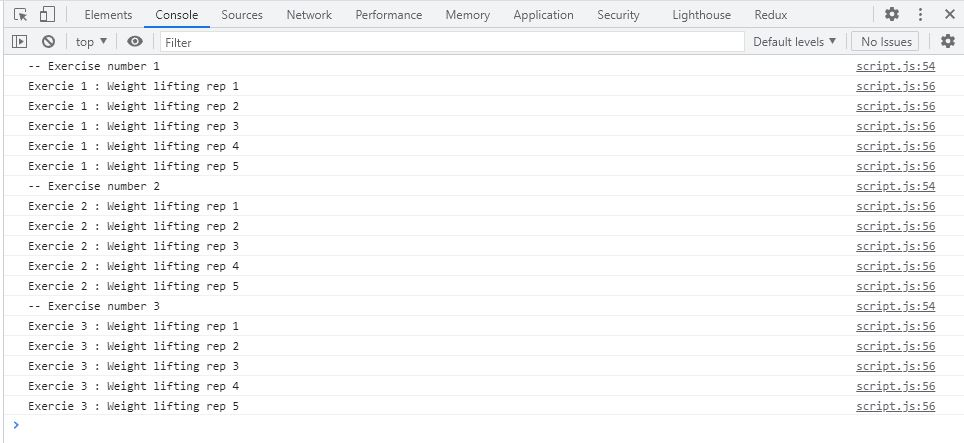# const - JS | lectureJavaScript Fundamentals 2

Looping Backwards & Loops in Loops

In this lecture, we will see how to loop backward in an array and inside another loop.

Let's consider our well-known user array.

script.js
``````const user = [
'John',
'Doe',
'Software Engineer',
32,
['Banana', 'Apple', 'Melon'],
['Eric', 'Ulrich', 'Mary'],
];``````

Looping backward means the first element we want to log will be `[Eric,...]`, `[Banana,...]`, `32`, and up to the value `John`. We looped from the beginning in the previous lecture starting from index 0 right up to index 5. But now, we want to start at index 5 down to 0.

script.js
``````/**
* The initial value of i should be 5 knowing that, it is the index of the
* last element of the array. Again, remember arrays are zero based.
*
* Knowing that we shouldn't hard code because that is a bad practice,
*
* 5 = user.length (6) - 1
*
* I hope the condition is clear enough. We have to stop at 0 because
* that is the index of the last element
*
* We previously updated the counter by doing i++ but now since we are
* going from the last index (5) to the first, we need to decrement
*/

for (let i = user.length - 1; i >= 0; i--) {
console.log(user[i]);
}``````Now that we have seen how to loop backward in an array let's see how to create a loop inside another loop. It might sound difficult, but it isn't.

Let's consider our weight lifting exercise again, which we had in the first loop lecture.

Let's say we have three exercises, and for each of those three exercises, we have to make five repetitions of weight lifting. To log all these exercises, we need a loop inside a loop.

script.js
``````for (let exercise = 1; exercise < 4; exercise++) {
console.log(`-- Exercise number \${exercise}`);
for (let rep = 1; rep < 6; rep++) {
console.log(`--- Weight lifting rep \${rep}`);
}
}``````In the console, what happens is that we start with `exercise = 1` we get `-- Exercise number 1` being logged in the console, then a new loop is created and executed, which then creates the string `--- Weight lifting rep ...` from 1 to 5. When the inner loop is over, the first iteration is then over, making the outer loop go to its second iteration logging `-- Exercise number 2`. At the second iteration, the inner loop gets executed again, logging the string five times, and when it's over, the outer loop goes to iteration number three logging `-- Exercise number 3`. Again, at the third iteration, the inner loop is executed five times again. When the inner loop is done, the outer loop tries to go to the next iteration, but it doesn't since the condition (`exercise < 4`) is not satisfied.

We then end up with a total of 15 repetitions. We can include the number of the current exercise in the string found in our inner loop.

script.js
``````for (let exercise = 1; exercise < 4; exercise++) {
console.log(`-- Exercise number \${exercise}`);
for (let rep = 1; rep < 6; rep++) {
console.log(`Exercie \${exercise} : Weight lifting rep \${rep}`);
}
}``````